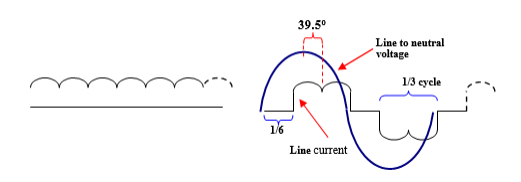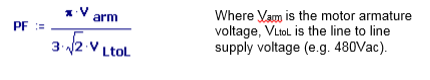1-317-844-7328

## AC vs DC Drives Advantages of Power Factor Comparison

Posted by Jason Justice on Mar 1st 2023

# Power Factor Comparison for AC and DC Drives

Most AC drives have an input power, full wave rectifier. These drives have a clear advantage over SCR based DC drives when the power factor for each is compared.

## Displacement Power Factor:

The displacement power factor is the ratio of the electrical power to the applied voltage times the current at the fundamental frequency (60 Hz). It does not account for higher harmonic frequencies. The power factor can also be determined from the cosine of the angle (displacement angle) between the voltage (line to neutral) and line current. This displacement power factor is what is measured by the power utility companies.

## Total Power Factor:

The total power factor takes into account the higher frequency harmonics and so is the ratio of the “real” power to total electrical power (RMS voltage times the RMS current). Although this could be considered to be a more accurate measure, it is presently not used in assessing power consumption costs.

## Displacement Power Factor for a DC Drive:

Most DC drives are three phase, 6 SCR, full wave converters, where the SCRs are gated at a place on each sinewave (of the line voltage) to produce the needed output voltage to the motor. As each sinewave progresses, the earlier each SCR is gated the higher the output voltage. Regardless of the output voltage, however, all the current to the motor has to intermittently flow in each of the three phase lines (see the illustration below). This means that if the motor is at some load (torque) level, the same current flows in the AC line whether the motor is at high or low speed. The only difference is the relative displacement between the voltage and current waveforms. Near zero speed a DC motor might be at full rated torque but because it is turning slowly, it will be producing nearly no mechanical power, and therefore the drive would be consuming very little electrical power. Nevertheless, the volt-ampere (VA) level would be just as high as if the motor was at maximum speed. Consequently when a DC drive is run at low speed, the power factor is always very low. A DC drive connected to a 480Vac line powering a motor at 500 Vdc will have a displacement power factor of approximately 77%. At lower speeds the power factor (PF) drops off proportional to the armature voltage, so that at half speed, or 250 V, PF = 39%, or at 10% speed, PF=8%.In the illustration above, the typical armature current is shown on the left. As SCRs are gated on, sections of the armature current flow in the AC line as shown on the right. For a 480Vac line and a motor running at 500Vdc, the center of the current waveform is delayed about 39.5 degrees from the peak of the line to neutral voltage waveform. For this case the power factor, PF = cos (39.5o) = .772

The displacement power factor for a DC drive running at any armature voltage can be found from:Note that this equation is just the ratio of armature voltage to average voltage from a 3 phase full-wave rectifier which is: (3/π) (√2 VLtoL).

## Displacement Power Factor for an AC Drive:

Most AC drives use a full wave diode bridge to rectify the incoming AC power to produce a DC bus. Because the current is drawn from the line when the voltage is at its peak, the line current is quite closely in phase with the line voltage. Although the current waveform contains a fair number of harmonics, the displacement power factor is near one – typically greater than 99%

Unlike that of the DC drive, not all the motor current needs to flow in the incoming lines. This is because the, so called, magnetizing portion of the total current for the motor is current that does little real work – it is current than flows between the DC bus in the drive and motor and requires very little current from the line to maintain it. The drive acts as power factor correction for the motor. Also if the motor is runs at lower than maximum speed the input line current drops off nearly proportional to the speed. At near zero speed, the motor can be producing rated torque but the required line current will be nearly zero. At low speed the motor is producing little mechanical power so only the power to supply motor and drive efficiency losses is required from the AC line.Mechanical Electrical Systems, Inc. is a Featured Supplier on IndustryNet